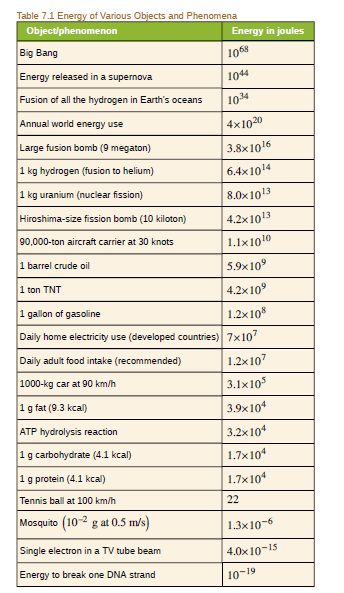Chapter 28, Problem 48PE

Chapter
Section
Textbook Problem

(a) Using data from Table 7.1, calculate the mass converted to energy by the fission of 1.00 kg of uranium. (b) What is the ratio of mass destroyed to the original mass, Δ m / m ?To determine

(a)

The fission of 1kguranium how many kilograms of mass are converted in to energy.

Explanation

Given info:

• The mass of uranium is 2.00×1031kg.
• fission of 1kguranium produces 8.00×1013J of energy.

Formula used:

Formula to find mass using Einstein's mass energy equation,

Δm=E2c

Here, Δmis the converted mass of star, Eis the energy produced and cis the speed of light.

Calculation:

Substituting the given values, we get,

Δm=(8

To determine

(b)

The ratio of the mass destroyed to the original mass.

Still sussing out bartleby?

Check out a sample textbook solution.

See a sample solution

The Solution to Your Study Problems

Bartleby provides explanations to thousands of textbook problems written by our experts, many with advanced degrees!

Get Started